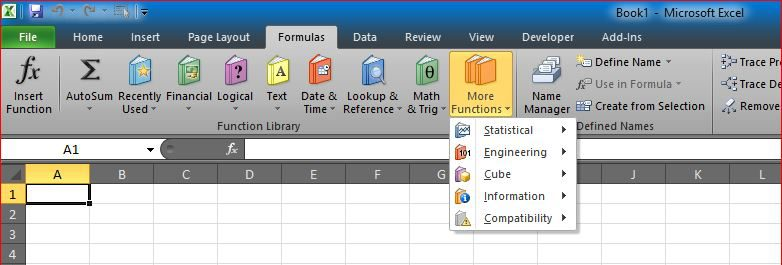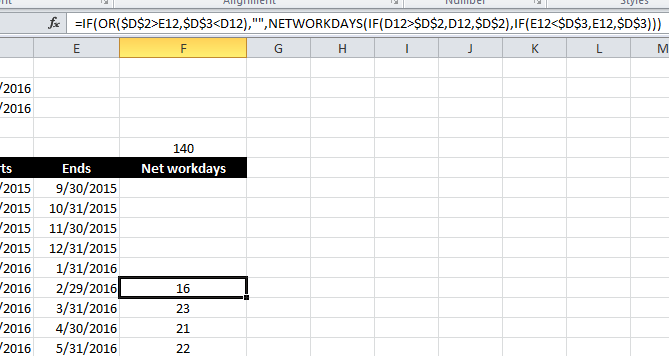# Workdays – Across monthsGet total number of days per month
This was a question from one of my friends, This set of formulas will calculate how many days (Networkdays) in each of the months in the list for a given Start and End dates

If Range of dates are across multiple years, user can copy columns C, D, E and F to fill those years

The months for the list are automatically populated starting from Jan of the year of the start date

The main formula in F7 should be:

```=IF(OR(\$D\$2>E7,\$D\$3<D7),"",
NETWORKDAYS(IF(D7>\$D\$2,D7,\$D\$2),IF(E7<\$D\$3,E7,\$D\$3)))```